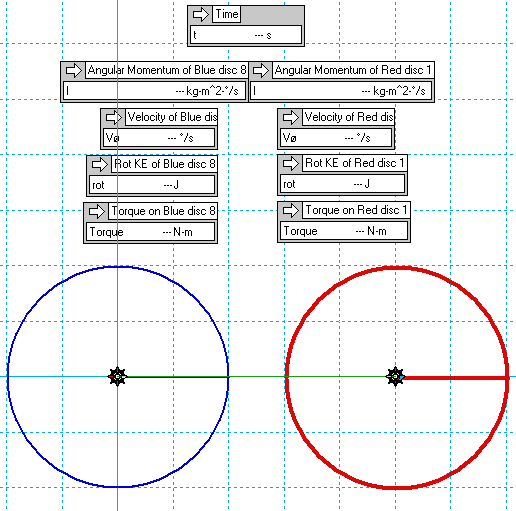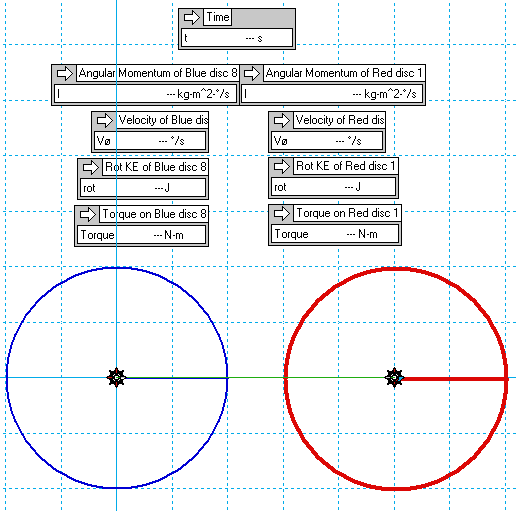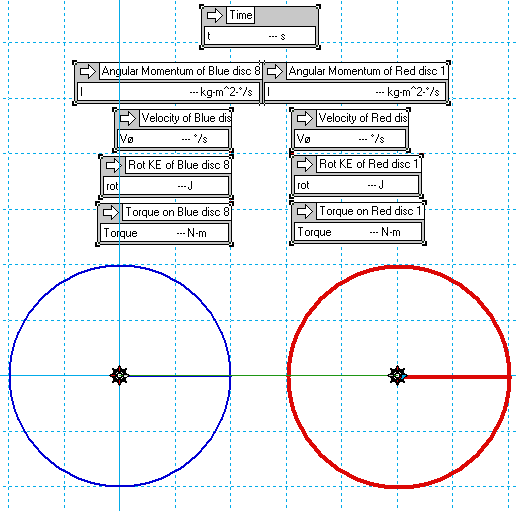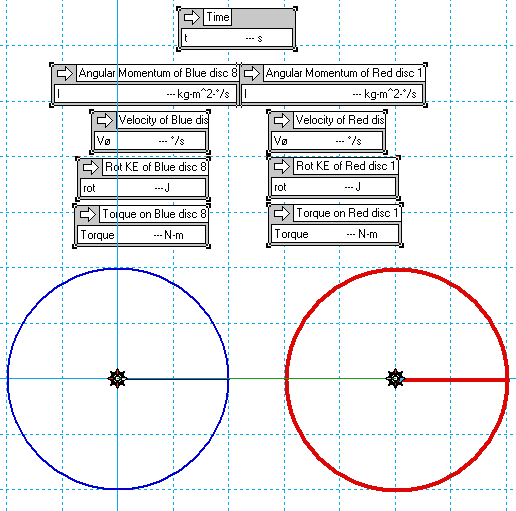# Non-dissipative RKE losses?

• B
• Mr Vibrating

#### Mr Vibrating

Two identical discs, one of which is rotating about its center, the other, stationary.

Suddenly they're locked / fused / meshed together (by whatever means - at 1:1 ratio).

The angular momentum is thus shared equally between them - one is accelerated, by the same amount the other is decelerated.

We still have all of our momentum, but each disc now only has a quarter the original energy, for a net total of half the original... the other half seems to have been factored out of existence by the spontaneous doubling of the MoI. If we began with 10 J of RKE on one disc, we now have 2.5 on each, and 5 J 'destroyed'.

Successive divisions will likewise eliminate another 50% total system energy per step. All of the momentum remains conserved, but its energy value keeps halving.

We can 'undissipate' the momentum, by adding a rotary spring that is wound up by one disc, braking it to a halt, then transferring this loaded spring over to the second disc, unloading into and thus further accelerating it. But this only causes the energy to sum - so we could add the 2.5 J from one disc back onto the other for a 5 J total. But the other 5 J we're missing doesn't spontaneously re-appear - so this loss mechanism isn't time-symmetrical, merely consolidating the remaining energy. Is this a manifestation of the 2nd law, or what? I understand where the energy's 'gone', and each disc always has the right KE for its given momentum, so i can see why we can't recover the original system KE, even though we can re-unify all of the momentum back into a single disc, from any number of discs it's been divided into. But i usually only encounter the 2nd law with regards to entropic losses, whereas here, the loss mechanism is non-thermal.

This appears to be a time-dependent input / output asymmetry, from a simple collision?

And it gets worse - suppose both discs had equal opposite angular momentum!? All momentum and KE destroyed!?

Or consider that one disc is simply spinning twice as fast as the other, but in the same direction - again, they're suddenly locked together, and again, all system momentum fully conserved (example: one is spinning 200°/s CW, the other 100°/s CW, after locking together each now has 150°/s CW), and yet some energy is still apparently 'obviated' out of existence.

Final example, reverse the sign of the above 200°/s disc, relative to the other one, and upon locking together, the CW and CCW momentums equalise to just 50°/s velocity on each disc - again, seemingly destroying momentum and energy.

Obviously, in a real collision some dissipative loss is inevitable but here I'm disregarding friction etc. precisely to focus on the apparent non-dissipative losses.

So is my interpretation / conclusions correct (and thus trivial, if surprising to me), or am i missing something?

I'll try posting up some examples from WM2D in a sec..

All these tests use the same 10 kg discs.

Each test begins with the discs uncoupled for the first 500 ms. Then the 1:1 transmission activates, and the sim continues for another 500 ms.

1234Two identical discs, one of which is rotating about its center, the other, stationary.

Suddenly they're locked / fused / meshed together (by whatever means - at 1:1 ratio).

The "means" makes all the difference. What you have here is a collision between two objects. If it is an elastic collision then momentum and energy is conserved. If it is an inelastic collision then only momentum is conserved.

Much of what you post suggests you misunderstand this.

We can 'undissipate' the momentum, by adding a rotary spring that is wound up by one disc, braking it to a halt, then transferring this loaded spring over to the second disc, unloading into and thus further accelerating it.

Using a spring would make it an elastic collision so energy would be conserved.

I understand where the energy's 'gone', and each disc always has the right KE for its given momentum, so i can see why we can't recover the original system KE, even though we can re-unify all of the momentum back into a single disc
.

That's not correct. If the collision is inelastic you cannot get back to the state where you have one disc with it's original momentum. That would mean it also has the original KE which isn't possible if some has left in the form of heat.

Obviously, in a real collision some dissipative loss is inevitable but here I'm disregarding friction etc. precisely to focus on the apparent non-dissipative losses.

I believe that's also a mistake. What do you mean by "non-dissipative losses"? Where does the energy go in an inelastic collision if it's not dissipated as heat?

So is my interpretation / conclusions correct (and thus trivial, if surprising to me), or am i missing something?

Thanks for taking the time, though I've now resolved it.

FWIW the collision is fully elastic - as explained, the area of interest is the non-dissipated missing energy.

Again, when the momentum of a rotating disc is suddenly coupled to an identical stationary one, that redistrubution of the system momentum results in half of it residing on each disc, yet now the net system energy is half its original.

So, if we had 100 Joules originally on the one disc, and then we share half of its momentum with a second, we now have 25 J on each, and 50 J has effectively disappeared from the system.

Obviously, a non-dissipative loss is one that does not transform energy to low-grade heat. This is not a frictional or entropic loss - all of the momentum remains conserved between the two discs, which wouldn't be possible if any energy had been dissipated.

So if this is an effective example of destruction of classical energy, then the question of its irreversibility naturally follows.

As such, when the energy of the two discs is transferred back to one, such as by applying a rotary spring, braking one to a halt and then transferring the loaded spring over to the other, what is transferred is of course energy, not momentum, and so the energy adds, not multiplying, and the new system momementum is 1.414 times its original, with the missing 50% system energy remaining unrecoverable.

The resolution is that it's trivial to exchange any quantity of energy for any other quantity of momentum; a given energy can correspond to a high, or low momentum.

Likewise, it is trivial to increase a system's MoI (such as by raising the mass or radius), but costs energy to reduce it.

So when we suddenly double the MoI, we divide the net RKE by two, writing half of the energy out of existence, but in order to spontaneously halve the MoI we'd need to re-input that missing 50% net energy.

As far as i can see, you could stop a freight train this way without converting a Joule to heat..

Non-dissipative loss mechanisms are rare, but do exist, and this appears to be such an example..

FWIW the collision is fully elastic - as explained, the area of interest is the non-dissipated missing energy.

Again, when the momentum of a rotating disc is suddenly coupled to an identical stationary one, that redistribution of the system momentum results in half of it residing on each disc, yet now the net system energy is half its original.

There is something inconsistent because the definition of an Elastic Collision is...

https://en.wikipedia.org/wiki/Elastic_collision

An elastic collision is an encounter between two bodies in which the total kinetic energy of the two bodies after the encounter is equal to their total kinetic energy before the encounter.

I believe the mistake you are making is to assume that after the collision they are both rotating. If all of the energy is transferred from one disc to the other then both conservation of momentum and conservation of energy is demonstrated.

Again, when the momentum of a rotating disc is suddenly coupled to an identical stationary one, that redistrubution of the system momentum results in half of it residing on each disc, yet now the net system energy is half its original.

So, if we had 100 Joules originally on the one disc, and then we share half of its momentum with a second, we now have 25 J on each, and 50 J has effectively disappeared from the system.
This coupling can only be accomplished by an inelastic collision. It may be more or less difficult to see how the "missing" energy has been dissipated, but if you correctly and completely analyze the interaction, you will always find that it has been dissipated as heat somewhere.

So if this is an effective example of destruction of classical energy...
It's not. However, it will take more complete specification of the exact mechanism to demonstrate why.

•CWatters
There is something inconsistent because the definition of an Elastic Collision is...

https://en.wikipedia.org/wiki/Elastic_collision

...thus implying that an 'inelastic' collision is one in which frictional / entropic / dissipative losses are present.

Here, they're disregarded. Friction is not included in the calculations, nor simulations.

Thus, we're assuming fully elastic, ie. fully conservative, interactions. Again, all of the momentum remains conserved in this first example - we begin with 150 kg-m^2-°/s on one disc prior to the collision, and end 75 kg-m^2-°/s on each after the collision. So we have a perfectly conservative equal and opposite exchange of momentum, with one disc decelerated by the same amount the other is accelerated.

But the energy value of that conserved momentum is now half its original, due to the division into a second equal MoI.

RKE = half angular inertia times angular velocity squared, and angular inertia = mass times radius squared, hence if we suddenly double the mass, as in this case, RKE is reduced by 50% - this energy has simply been obviated by a spontaneous reshuffling of the distribution of M and V for a given conserved P.

In other words, the energy value of a given momentum is entirely dependent upon its specific distribution of mass and velocity, which are covariant due to the conservation of momentum. As such, when mass doubles, velocity must halve, and KE - evolving as half the square of velocity - thus drops fourfold. Accordingly, each disc ends up with just a quarter of the initial system energy, for a net total of half the original. The remainder no longer exists..

...thus implying that an 'inelastic' collision is one in which frictional / entropic / dissipative losses are present.

Here, they're disregarded. Friction is not included in the calculations, nor simulation ions.

Thus, we're assuming fully elastic, ie. fully conservative, interactions...

As has been pointed out already, if the interaction is fully elastic the two disks will not behave as your simulation assumes. If the two disks behave as your simulation assumes, then the interaction is necessarily inelastic and you can't just assume otherwise.